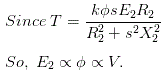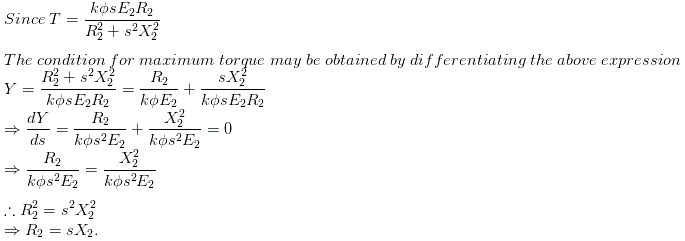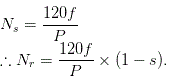# MCQs on Electrical Machines

##### Page 94 of 114. Go to page 1 2 3 4 5 6 7 8 9 10 11 12 13 14 15 16 17 18 19 20 21 22 23 24 25 26 27 28 29 30 31 32 33 34 35 36 37 38 39 40 41 42 43 44 45 46 47 48 49 50 51 52 53 54 55 56 57 58 59 60 61 62 63 64 65 66 67 68 69 70 71 72 73 74 75 76 77 78 79 80 81 82 83 84 85 86 87 88 89 90 91 92 93 94 95 96 97 98 99 100 101 102 103 104 105 106 107 108 109 110 111 112 113 114
01․ The torque developed in IM is nearly proportional to
1 / V.
V2.
V.
1 / V2.02․ In case of 3 - ÃŽÂ¦ IM, plugging means
pulling the motor directly on line without a rotor.
locking of rotor due to harmonics.
interchanging two supply phases for quick stopping.
starting the motor on load which is more than the rated load.

An IM can be quickly stopped by simply inter changing any of its two stator leads. It reverses the direction of the rotating flux which produces a torque in the reverse direction, thus applying brake on the motor, this is called plugging.

03․ When R2 is rotor resistance, X2 the rotor reactance at supply frequency and s the slip, then the condition for maximum torque under running condition will be
sR2 = X2.
R2 = s2X2.
R2 = s2X2.
R2 = sX2.04․ The rotor electromotive force of the IM is observed to make 100 complete alterations per minute, calculated slip
3.33 %.
3 %.
0 .001 %.
none of the above.

100 alterations per minute = 100 / 60 cycles/sec. sf = 1.6667 Hz. ∴ s = 1.6667 / 50 = 3.33 %.

05․ Which of following methods are suitable for the speed control of squirrel cage induction motor? 1. Voltage control. 2. Frequency control. 3. Pole changing method. 4. Rotor resistance control select the correct answer using the codes given below:-
2, 3 and 4.
1, 2 and 3.
1, 3 and 4.
2 and 4.

Since rotor resistance control is suitable only for woumd rotor induction motors.

06․ In a star - delta starting of an IM
reduced voltage is applied to the stator.
applied voltage per stator phase is 57.7 % of a line volt
line voltage is 57.7 % of stator voltage.
resistance is inserted in the rotor.

When the motor is star connected star, the applied voltage over each phase is reduced to ( 1 / √3 ) times of the supply voltage. ∴ Line phase voltage is 57.7 % of line voltage.

07․ A 3 - φ induction motor can also be referred to as a
asynchronous motor.
transformer.
repulsion motor.
shunt motor.

The rotor of a 3 - φ IM can never run at synchronous speed. Because at synchronous speed the flux cutting between the stator and rotor is zero leading to no torque production, thus bring the rotor speed immediately below synchronism.

08․ Which of the following methods of speed control is not affected through stator side?
Change of rotor resistance.
Change of number of pole.
Change of supply voltage.
Change of supply frequency.

The rotor resistance can only be added onto the rotor side and the other three modifications can be done from the stator side.

09․ The wound rotor type IM is used for____________ speed operation.
constant.
maximum.
minimum.
variable.

In this type of IM, the rotor has the same number of poles as the stator and the windings are made of wire, connected to slip rings on the shaft. Carbon brushes connect the slip rings to an external controller such as a variable resistor that allows changing the motors slip rate, by this motor can obtain variable speed.

10․ The rotor speed of an induction motor is given by
Ns = 120f / p Ãƒâ€” ( 1 - s ).
Nr = 120f / p Ãƒâ€” (1-s).
Ns = 120f /p Ãƒâ€” ( 1 - r).
none of the above.

The rotor speed of an IM is given by Nr = ( 1 - s)Ns.It is seen that the motor speed can be changed by change in frequency f, number of poles P, or slip s.

<<<9293949596>>>